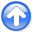First-break traveltime tomography with the double-square-root eikonal equationNext: Numerical Implementation Up: Theory Previous: DSR eikonal equation

## DSR tomography

The first-break traveltime tomography with DSR eikonal equation (DSR tomography) can be established by following a procedure analogous to the traditional one with the shot-indexed eikonal equation (standard tomography). To further reveal their differences, in this section we will derive both approaches.

For convenience, we use slowness-squaredinstead of velocityin equations 1, 3 and 4. Based on analysis in Appendix A, the velocity modeland prestack cubeare Eulerian discretized and arranged as column vectorsof sizeand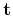of size. We denote the observed first-breaks by, and useandwhenever necessary to discriminate betweencomputed from shot-indexed eikonal equation and DSR eikonal equation.

The tomographic inversion seeks to minimize the(least-squares) norm of the data residuals. We define an objective function as follows:(5)

where the superscriptrepresents transpose. A Newton method of inversion can be established by considering an expansion of the misfit function 5 in a Taylor series and retaining terms up to the quadratic order (Bertsekas, 1982):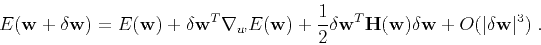(6)

Hereand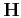are gradient vector and Hessian matrix, respectively. We may evaluate the gradient by taking partial derivatives of equation 5 with respect to, yielding(7)

whereis the Frechét derivative matrix and can be found by further differentiatingwith respect to.

We start by deriving the Frechét derivative matrix of standard tomography. Denoting(8)

as the partial derivative operator in the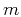's direction, equation 4 can be re-written as(9)

Here we assume that there are in totalshots and usefor first-breaks of theth shot. Applyingto both sides of equation 9, we find(10)

Kinematically, eachcontains characteristics of theth shot. Because shots are independent of each other, the full Frechét derivative is a concatenation of individual, as follows:(11)

Inserting equation 11 into equation 7, we obtain(12)

where, similar to,stands for the observed first-breaks of theth shot.

Figure 3 illustrates equation 12 schematically, i.e. the gradient produced by standard tomography. The first step on the left depicts the transpose of theth Frechét derivative acting on the correspondingth data residual. It implies a back-projection that takes place in theplane of a fixed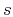position. The second step on the right is simply the summation operation in equation 12.cartonstd
Figure 3.
The gradient produced by standard tomography. The solid curve indicates a shot-indexed characteristic.To derive the Frechét derivative matrix associated with DSR tomography, we first re-write equation 1 with definition 8(13)

whereandareat sub-surface source and receiver locations, respectively. Note that in equation 13appears twice. Thus a differentiation ofwith respect tomust be carried out through the chain-rule:(14)

We recall thatandare of different lengths. Meanwhile in equation 13, bothandhave the size of. Clearly in equation 14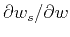and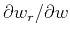must achieve dimensionality enlargement. In fact, according to Figure 1,andcan be obtained by sprayingsuch thatand. Therefore,andare essentially spraying operators and their adjoints perform stackings alonganddimensions, respectively.

In Appendix B, we prove thathas the following form: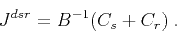(15)

Combining equations 7 and 15 results in(16)

Note that unlike equation 12, equation 16 can not be expressed as an explicit summation over shots.

Figure 4 shows the gradient of DSR tomography. Similarly to the standard tomography, the gradient produced by equation 16 is a result of two steps. The first step on the left is a back-projection of prestack data residuals according to the adjoint of operator. Becausecontains DSR characteristics that travel in prestack domain, this back-projection takes place inand is different from that in standard tomography, although the data residuals are the same for both cases. The second step on the right follows the adjoint of operatorsandand reduces the dimensionality fromto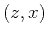. However, compared to standard tomography this step involves summations in not onlybut also.cartondsr
Figure 4.
The gradient produced by DSR tomography. The solid curve indicates a DSR characteristic, which has one end in planeand the other in plane. Compare with Figure 3.First-break traveltime tomography with the double-square-root eikonal equationNext: Numerical Implementation Up: Theory Previous: DSR eikonal equation

2013-10-16